University of Utah § Department of Mathematics

 High School Program Lecture

# The Geometry of Perspective Drawing on the Computer

• Andrejs Treibergs
• University of Utah
• Department of Mathematics
• 155 South 1400 East, Rm. 233
• Salt Lake City, UTAH 84112-0090

Abstract. We present some mathematical ideas that occur in art and computer graphics. We touch upon the geometry of similar triangles, rigid motions in three space, perspective transformations, and projective geometry. We discuss computations behind rendering objects in perspective. We then describe vanishing points, answer how to measure distance in a receding direction in a perspective drawing and why a circle in three space becomes an ellipse when drawn in perspective. We use MAPLE generated computations and graphics to illustrate the ideas.
``I freely confess that I never had a taste for study or research either in physics or geometry except in so far as they could serve as a means of arriving at some sort of knowledge of the proximate causes...for the good and convenience of life, in maintaining health, in the practice of some art...having observed that a good part of the arts is based on geometry, among others the cutting of stone in architecture, that of sundials, and that of perspective in particular.´´
Girard Desargues

##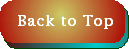Introduction.

Artists now make amazing images using computers (e.g., visit PIXAR.). But taking into account the complexity of all the physical laws of light requires extensive computation. We'll focus on mathematical concepts and derive the basic formulas that are at the heart of all rendering machines. We shall concentrate on the geometry of drawing objects, which can be described by points in space. We'll calculate the perspective transformations that locate the points on the drawing. Then we'll use MAPLE's capability to draw polygons and lines to show the effect of transformation. We'll apply this to show some mathematical / artistic concepts. (This note is a slightly expanded version of lecture notes [TA].)

A mathematical theory of perspective drawing could only be developed when the Renaissance freed painters to depict nature in a way closer to what they observed [IW]. The biographer Vasari (1511-74) says that the Florentine architect Filipo Brunelleschi (1337-1446) studied Greek geometry, developed a theory of perspective and undertook painting just to apply his geometry [KM]. The first treatise, Della pittura(1435) by Leone Battista Alberti (1404-72) furnished most of the rules. Our diagram of the perspective view of the circle occurs in his text. A complete mathematical treatment De prospectiva pingendi (1478) was given by the Italian fresco painter Piero della Francesca (1410-1492). Leonardo da Vinci (1452-1519) incorporated geometry in his painting and wrote a now lost text on perspective Tratto della pittura. Albrecht Dürer (1471-1528) also wrote a text on the practice of geometry Underweysung der Messung mid dem Zyrkel und Rychtscheyd (1525) which was important in passing on to the Germans the Italian knowledge of perspective drawing. In it, Dürer invented several drawing machines to teach perspective. Alberti was first to ask if two drawing screens are interposed between the viewer and the object, and the object is projected onto both resulting in two different pictures of the same scene, what properties do the two pictures have in common [KM2].

Alberti's Problem: What do the two projections have in common?

This question prompted the development of a new subject, projective geometry whose exponent was Girard Desargues (1591-1661). Desargues studied perspective geometry from a synthetic point of view, meaning he built up the geometry from axioms about points, lines and planes. A sampling is given in the section on projective geometry. There we address the question why the perspective image of a circle necessarily the ellipse. It can also be answered using analytic geometry methods, such as in our chapter on analytic geometry, where first, points and lines are reduced to equations. A modern deductive footing for perspective drawing was given later by Brook Taylor (1685-1731) and J. H. Lambert (1728-77). A competing point of view has held by mathematicians such as René Decartes (1596-1650), Pierre de Fermat (1601-1665) and Julius Plücker (1801-1868) who studied these question algebraically. Their work spurred the development of algebraic geometry. Mathematical issues and history are more completely covered in [BP], [DH], [FP], [M], [OW], [PD], [SD] and [WC].

Some current popular drawing texts such as Edwards' [EB] de-emphasize the analytical approach in favor of an intuitive sighting method for perspective. However, complicated drawing situations require more analysis [EB], [EM]. If a student wishes to pursue linear perspective in the history and art see, e.g. [CA]. There are a number of web sites worth perusing, [DJ], [EM], [MS] as are sites discussing perspective and the internet [KJ], [XX]. The computer science of graphics is discussed in [BP], [FP], [PP]. Many of our illustrations were generated by the MAPLE symbolic algebra, graphics and computation system. Our code in the MAPLE programming language is available at http://www.math.utah.edu/~treiberg/HS.html Differential geometric graphic applications of MAPLE are described in Oprea [OJ] and Rovenski [RV] and of MATHEMATICA in Gray [GA]. Other graphics packages to render differential geometric objects, e.g., are Richard Palais' 3D-Filmstrip or Konrad Polthier's JavaView.

#Parallel transformation of points.

The perspective transformations that describe how a point in three space is mapped to the drawing plane can be simply explained using elementary geometry. We begin by setting up coordinates. A projection involves two coordinate systems. A point in the coordinate system of an object to be drawn is given by X=(x,y,z) and the corresponding in the imaging system (on the drawing plane) is P=(u,v). If we use the standard right handed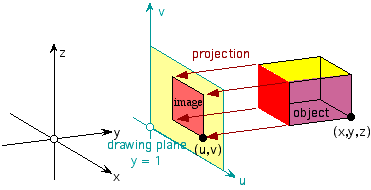Projecting an object to the drawing plane.

system, then x and y correspond to width and depth and z corresponds to height. On the drawing plane, we let u be the horizontal variable and v the vertical.

We can measure the distances between pairs of points in the usual way using the Euclidean metric. If
X1 = (x1, y1, z1) and P1 = (u1, v1) and so on, then

dist(X1, X2) = {(x1 - x2)2 + (y1 - y2)2 + (z1 - z2)2}1/2,

dist(P1, P2) = {(u1 - u2)2 + (v1 - v2)2}1/2

The projection from X to P is called a parallel projection if all sets of parallel lines in the object are mapped to parallel lines on the drawing. Such a mapping is given by an affine transformation, which is of the form

= f(X) = T + AX

where T is a fixed vector in the plane and A is a 3 x 2 constant matrix. Parallel projection has the further property that ratios are preserved. That is if X1, X2, X3 and X4 are collinear points in the object, then the ratio of distances is preserved under parallel projection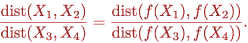Of course denominators are assumed to be nonzero.

To illustrate, let's begin with an object in three space, say a simplified house. It consists of the points [0,0,0], [0,0,3], [3.5,0,5], [7,0,3], [7,0,0], [0,9,3], [0,9,0], [7,9,3], [7,9,0], [3.5,9,5] which define eight corners of a box and two gable points and [3,9.1,0], [5,9.1,0], [5,9.1,8], [3,9.1,8], [3,10.2,0], [5,10.2,0], [5,10.2,8], [5,10.2,8] which define the chimney.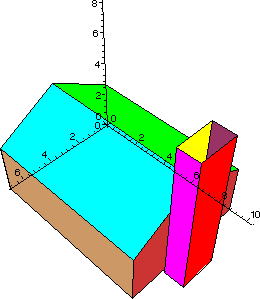MAPLE generated 3d plot of house.

The most frequent parallel projections are called elevations, oblique projections and isometric projections. The elevations are just the front, top and side views of the object. Thus the projections are given by the functions

Ffront(x,y,z)=(x,z),        Fside(x,y,z)=(y,z);       Ftop(x,y,z)=(x,y)

Applied to the house object, we get three views.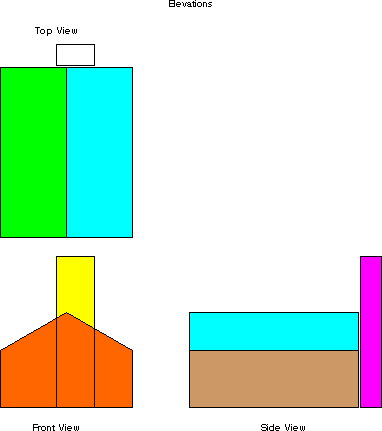MAPLE generated elevations.

In oblique projection, which is also called Cavalier projection, the front view is undistorted, but the sections of the object corresponding to y = y0 constant are drawn up and to the right depending on how far back y0 is. If we write w=(2-1/2, 2-1/2)T, the unit vector in the plane at 45°, then we may write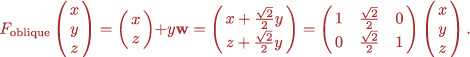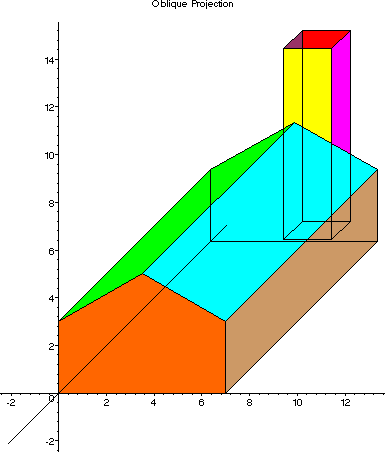The vectors have been written in column form to here to facilitate matrix multiplication, but we'll not fuss about whether a vector is a row or column and use both forms interchangeably. Note that since w is a unit vector, lengths in the y directions are mapped to equal lengths along the 45° line in the drawing. Indeed, putting c = 2-1/2,

dist((x0,y1,z0),(x0,y0,z0)) = |y1 - y0|;
dist(F(x0,y1,z0),F(x0,y0,z0))
= dist((x0 + c y1, z0 + c y1), (x0 + c y0,z0 + c y0))
= {(c y1 - c y0)2 + (c y1 - c y0)2}1/2 = |y1 - y0|.
Of course horizontal and vertical lines also preserve measurement. There is nothing special about 45° except that it is common. One can find drafting paper that has horizontal, vertical and 45° lines used to make oblique projections. Any unit vector w will do as well.

#Rotation of Vectors

Let R denote a rotation in the plane which moves points (x,y) about the origin an angle h0. If a vector is written in polar coordinates (x,y)=(r cos h,r sin h) where r={x2 + y2}1/2 is the distance of the point to the origin and h is the angle from the positive x-axis, dist((0,0),(x,y)), and h is the angle, then using trigonometric identities the rotated point

R(x,y) = (r cos(h0 + h), r sin(h0 + h))
= (r cos h0 cos h - r sin h0 sin h, r sin h0 cos h + r cos h0 sin h)
= (x cos h0 - y sin h0, x sin h0 + y cos h0)
has the same distance from the origin and a new angle h+h0. Bob Palais has a nice way of seeing this  [PR http://www.math.utah.edu/~cherk/ccli/bob/Rotation/Rotation.html] and we present a modification of his approach. Without knowing that sines and cosines are involved, it's possible to write down the rotation transformation just knowing what vectors are rotated into. For example, if we are given a vector (a,b) and wish to rotate it into the vector (0,r), then we know that r={a2+b2}1/2 is the length of the vector, and the angle of rotation has to be h0 where sin h0=a/r and cos h0 = b/r. Thus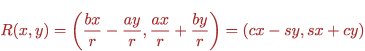Hence R(a,b)=(0,r) as desired.

A variant of oblique projection is called military projection. In this case the horizontal sections are isometrically drawn so that the floor plans are not distorted and the verticals are drawn at an angle. The military projection is given by rotation in the x-y plane and a vertical translation an amount z. Thus

Fmilitary(x,y,z) = ( c x - s y, s x + c y + z).

We chose h0=-53.1° so that c=0.6 and s=-0.8. Note that the floorplan is drawn rotated but without distortion.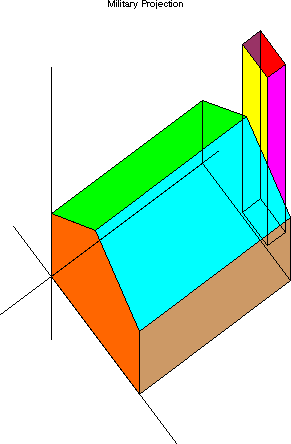MAPLE generated military projection.

The isometric projections are that class or parallel projections for which a round sphere projects to a round circle. The most common case is when measurements along the x-axis are plotted at 30°, those along the y axis at +150° and the vertical axis. Thus if w1=(31/2/2,1/2), w2=(-31/2/2,1/2) and w3=(0,1) are unit vectors at -30°, +30° and 90° (vertical), the isometric projection is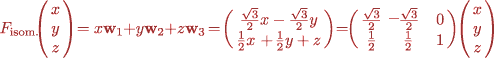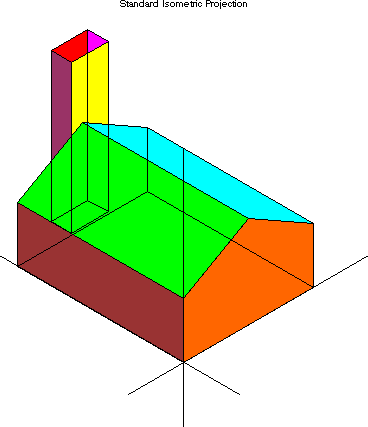MAPLE generated standard isometric projection.

The general parallel projection is obtained by applying a general affine transformation of the form F(X) = AX + T. If we choose T=0 and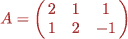and measure the depth according to GH(x,y,z) = x + 2y - z and then the picture is distorted so that none of the directions measure actual length. As mentioned before, parallel lines and proportions are preserved.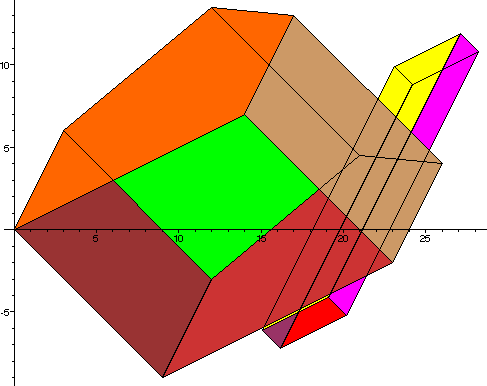MAPLE generated projection by the affine function F(X) = AX + T.

##Perspective projections.

We now describe the perspective transformation. It is the composition of a rigid motion followed by the perspective transformation that reduces distant objects. The rigid motion moves the object in front of the drawing plane in such a way that the eye point ep=(xe, ye, ze) is moved to the origin, so that the vector from eyepoint to centerpoint cp=(xc, yc,zc) toward which the eye is looking is moved to the positive y-axis and so that the vertical line through the centerpoint is drawn vertical. We shall accomplish the rigid motion by first translating the object to move the eyepoint to the origin using

T(x,y,z)=(x - xe, y - ye, z - ze).

Let the new vector eye to center be the displacement dp:=T(cp). Then we rotate the object around the origin. Every rotation is the composition of a rotation around the z-axis by an angle h, around the new x-axis by an angle k and around the y-axis by an angle l. The three angles h, k, k are called the Euler angles. We only need the first two rotations, and we can compute the cosines and sines involved using only the eyepoint and centerpoint coordinates. First we rotate dp around the z-axis so that (dp1, dp2) moves to (0, r1) where
r1 = {dp12 + dp22}1/2. Letting s1 = dp1/r1, c1 = dp2/r1 then the rotation is as before

R( x, y, z) = (c1 x - s1 y, s1 x + c1 y, z).

Let rdp= R(dp)=(0, rdp2, rdp3) be the rotated dp. The length of rdp is the same as the length of dp which is r2 = {dp12 + dp22 + dp32}1/2 = {rdp22 + rdp32}1/2. The second rotation takes rdp to (0, r2, 0). Setting c2 = rdp2/r2, s2 = rdp3/r2, the rotation around the x-axis becomes

S( x, y, z) = (x, c2 y + s2 z, - s2 y + c2 z).

The composite SRT(X) = S(R(T(X))) is the desired rigid motion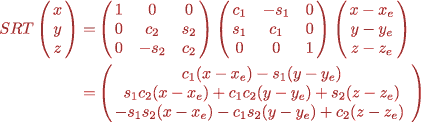The perpendicular projection is the front view or (x,z) part of the rotated object

Fperp.(x,y,z)= [ c1(x-xe) - s1(y - ye), -s1 s2(x - xe) - c1 s2(y - ye) + c2(z - ze)]

and the depth is computed by GPpP(x, y, z)= s1 c2(x - xe) + c1 c2(y - ye) + s2(z - ze). For example, taking the eyepoint ep=(11.0,-15.0,2.0) and centerpoint cp=(3.5,5.0,3.0) projects the house so: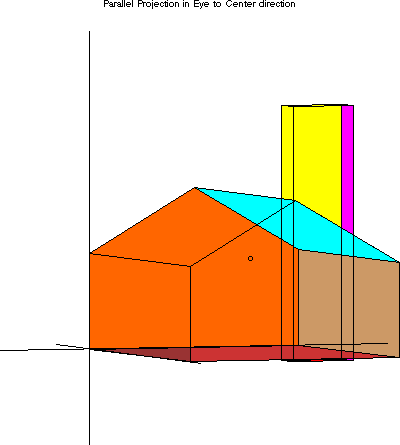MAPLE generated orthogonal projection.

Because light reflecting off the object travels in straight lines, the object point is seen on the drawing plane at the point where the line from the eyepoint to the object point intersects the drawing plane. The perspective transformation is simply to deduce the coordinates (u,v) on the drawing plane, which is a distance d from the origin, from the point X=(x,y,z) using triangles. The triangles (0,0):(0,d):(u,d) and (0,0):(0,y):(x,y) in the x-y-plane and the triangles (0,0):(d,0):(d,v) and (0,0):(y,0):(y,z) in the y-z-plane are similar. It follows that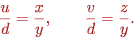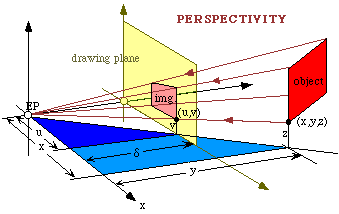Similar triangles used in computing perspective projection.

We have been using d=1 from which the perspective transformation may be calculated.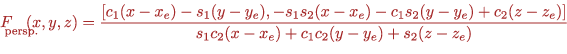This is just the x-z-coordinates of the perpendicular transformation divided by the depth (y-coordinate.) Using the same eyepoint and centerpoint as for the perpendicular transformation, we plot the house by perspective transformation.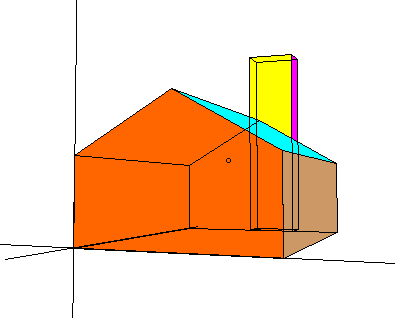MAPLE generated perspective projection.

Perspective transformations have the property that parallel lines on the object are mapped to pencils of lines passing through a fixed point in the drawing plane. To see this, note that each line in the rotated object lies in the plane passing through the line and through the eyepoint. This plane intersects the drawing plane in a line hence the image of a line in space is a line in the drawing. Any parallel lines in the object are parallel to the drawing plane or not. If the lines are parallel to the drawing plane (the y-coordinates on the line are constant) then the division by the depth (the y coordinate of the rotated object) is division by constant. Thus the formula reduces to a constant multiple of the numerator which is an affine transformation that maps parallel lines to parallel lines. If the parallel lines are not parallel with the drawing plane, then their image on the drawing plane passes through a fixed point, called the vanishing point. The easiest way to see this is to consider a pair of points on two parallel lines that travel together away from the drawing plane. Imagine that a wire of fixed length connects the points. Because the pair can get farther and farther from the drawing plane without letting go the wire, their perspective images get closer and closer in the drawing since the denominators are getting large whereas the difference in their (x,z) directions are bounded. Imagine the cliché of two rails of a track converging at infinity.

For general choices of the eyepoint and centerpoint, the parallel lines originally in the x, y and z-axis directions are not rotated to a position parallel to the drawing plane. Thus these three directions each have their own vanishing points. This is called three-point perspective. The three points may not so easily seen since they may not be within the cone of vision that limits the width of our view. To illustrate one and two point perspective we change our eye and center points to guarantee some parallel lines parallel to the drawing plane.

##One-point perspective.

Let us consider specific choices of eyepoint and centerpoint for which some of the objects axes are parallel to the drawing plane. Let the eyepoint ep=[6.0,-15.0,2.0] and the centerpoint cp=[6.0,5.0,2.0]. Because dp=[0,20,0] no rotation is necessary. The x and z-axes are parallel to the y=1 plane. The perpendicular projection is just the front elevation and the perspective view has one vanishing point corresponding to the y-axis direction. The vanishing point is indicated (it is the position of the centerpoint.)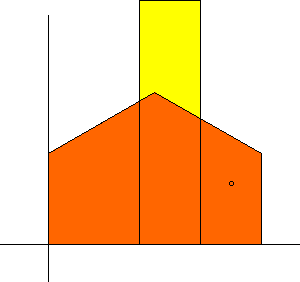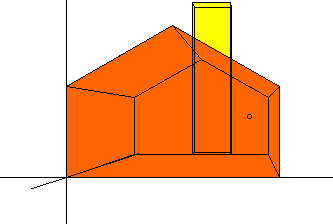MAPLE generated front elevation and one point perspective projection.

##Two-point perspective.

Let the eyepoint ep=[16.0,-15.0,2.0] and the centerpoint cp=[6.0,5.0,2.0]. This time dp=[-10,20,0] so that the only rotation is about the z axis. The z-axis is parallel to the y=1 plane. The perpendicular projection is now a corner elevation and the perspective view has two vanishing points corresponding to the x- and y-axis directions. The centerpoint is indicated.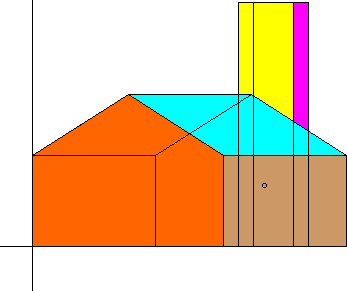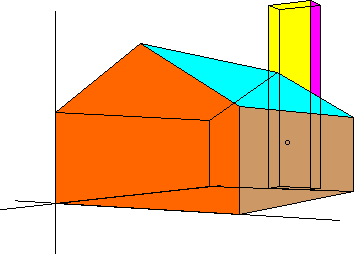MAPLE generated orthogonal and two-point perspective projection.

Another pair of views come by taking the eyepoint ep=[-6.0,5.0,9.0] and the centerpoint cp=[6.0,5.0,2.0]. This time the horizontal lines are parallel to the drawing plane but the vertical and receding lines are not. Therefore the vanishing points correspond to the vertical and receding directions.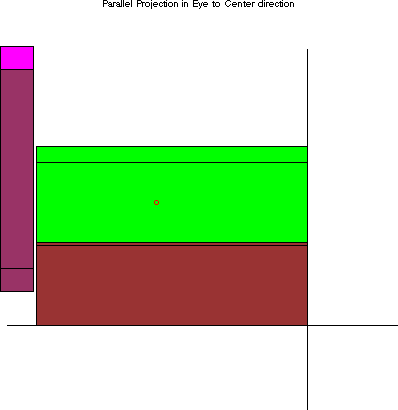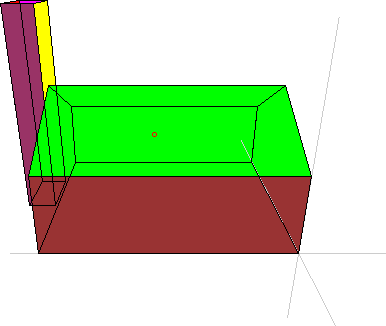Another MAPLE generated orthogonal and two-point perspective projection.

##Using vanishing points and measuring points.

The maximum view that the eye can take in is a cone of about 30° about its axis (the cone of vision.) It is possible for the computer to plot points outside the cone of vision, but such a drawing has a distortion like a fisheye camera photo. Thus usually both vanishing points aren't visible in the same scene, as in this computer-generated view of a cube with parallel lines.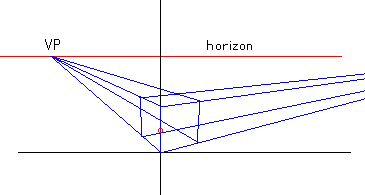MAPLE generated perspective view of unit cube showing vanishing points.

How do we locate the vanishing points in the drawing? The vanishing points for the x-axis and y axis parallels are always on the horizon line. If d is the distance from eye to drawing, then the two vanishing points in the drawing for x-axis and y-axis lines are on lines which meet at the eyepoint at 90°. This is easiest to see by imagining the top view.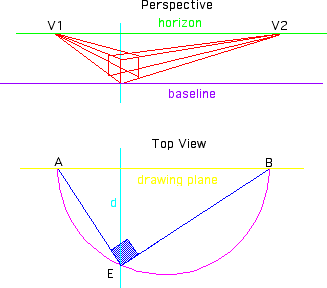MAPLE generated perspective view and construction of vanishing points from top view.

The drawing plane is a distance d from the eyepoint E. The rays emanating from the eyepoint at right angles parallel to the y and x-axes are the line segments EA and EB. A is the u-coordinate of the y-axis vanishing point V1 and B is the u-coordinate of the x-axis vanishing point V2. The v-coordinates are v=0 which corresponds to the eyelevel and horizon line. A circle whose center is on the drawing line and passes through the eyepoint intersects the drawings line at two points, say A and B for which AEB is a right angle. This is the geometric fact that a diameter AB subtends an angle 90° from any point E on the arc AEB.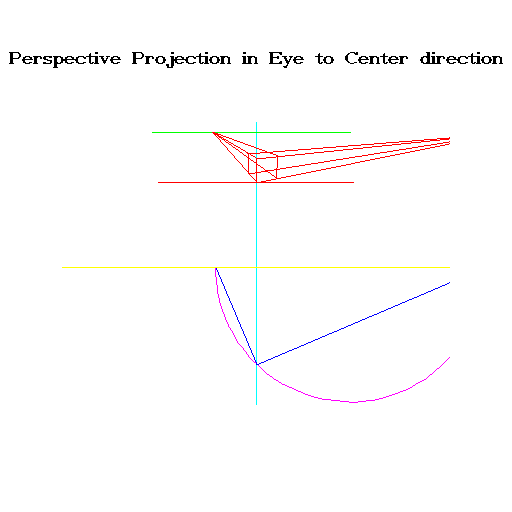MAPLE generated perspective and top view of vanishing points and their construction.

How do we measure distances in the receding direction? The idea is to figure out sets of parallel lines which transfer measurements along the baseline, a line parallel to the drawing plane, to the receding line. The projective transformation may scale but not distort distances along the baseline. To see how this works, consider the top view of a 3 x 3 square.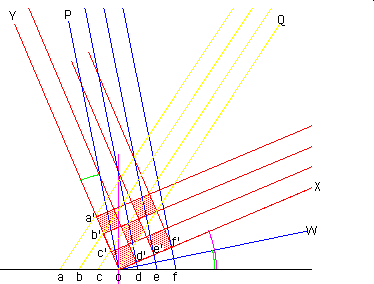MAPLE generated parallel sets of measuring lines.

The baseline is the line af. The baseline has equally spaced points a, b, c, o, d, e, f in order. The spacing is the same as along the square o, c', b' a' and o d', e', f'. The square has been rotated an angle foX. The parallels to oX and the parallels to oY are along the two sides of the square. Their perspective images converge to two vanishing points. The other two sets of lines are called measuring lines. One family are the parallels oP, dd', ee', ff' measure the oX side of the square and the other set of parallels oQ, cc', bb', aa' measure the oY side of the square. This is what it looks like in perspective.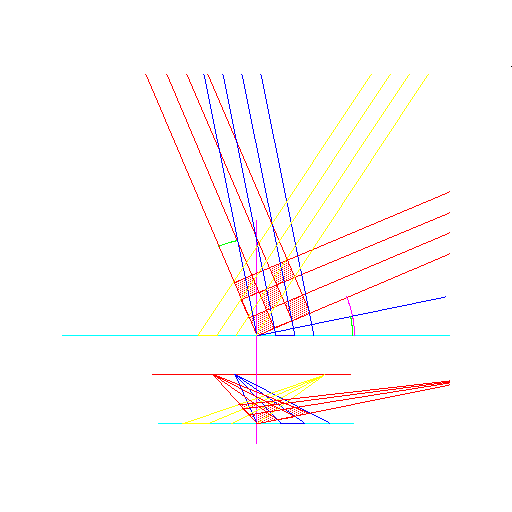MAPLE generated top and perspective views showing parallel measuring lines, vanishing and measuring points.

Because the lines connect equally spaced points, the triangles fof' and aoa' are isosceles. This means that if the line oW is chosen so that the angle foW bisects the angle foX, then the lines oP and oW are perpendicular and the angle Similarly, the triangle aoa' is isosceles so the angle But since the total angle of a triangle is and since they are supplementary, It follows that so These angles may be easily constructed on the circle.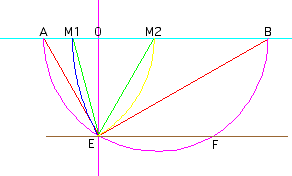MAPLE generated top view for constructing vanishing points and measuring points.

As before, we locate the eyepoint E and centerpoint O on the drawing and let line EF be parallel to AB. The sides of the box from the previous diagram are along the rays EA and EB so that the vanishing points in the drawing are located at A and B. Since a line intersects parallel lines so that opposite angles are equal, Draw a circular arc with center B and radius BE until it meets the drawing plane line AB at M1. EBM1 is a similar triangle to fof' so Thus M1 is the point where the eye views the first family of measuring parallels; thus M1 is the vanishing point for this set of parallels. Similarly, so that if one draws a circle with center A and radius AE then this circle intersects the picture plane line at M2. Now the angle so that the point M2 is the vanishing point for the second family of measuring lines. Now we can use the measuring lines to mark off equispaced points on the perspectively receding lines.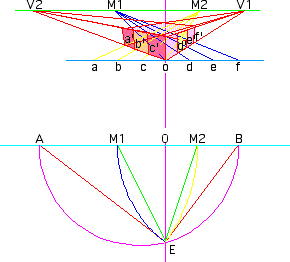MAPLE generated measuring lines viewed in perspective and their construction viewed from the top.

We mark off equally spaced points a-f on the baseline as before. The lines oV1 and oV2 correspond to the bottom edges of the box. Moving up one unit from o gives the upper corner of the box and the rays to V1 and V2 give the upper front edges. Now, the first measuring family was chosen so that the intersections with the right front edge were points spaced the same distance apart as on the baseline. Thus, where the lines dM1, eM1 and fM1 intersect oV1 are the equally spaced points d', e' f' in the perspective drawing. Similarly, the measuring family of parallel lines for the left side of the box have a vanishing point at M2. The intersection of aM2, bM2 and cM2 with oV2 correspond to the equally spaced points a', b', c' on the line oV2. The rest of the box is constructed by extending the vertical lines up from a'-f'. If one is using two-point perspective, these are truly vertical in the u-v-plane. Otherwise you have to use the vanishing point corresponding to the vertical family (which is probably way below the picture.)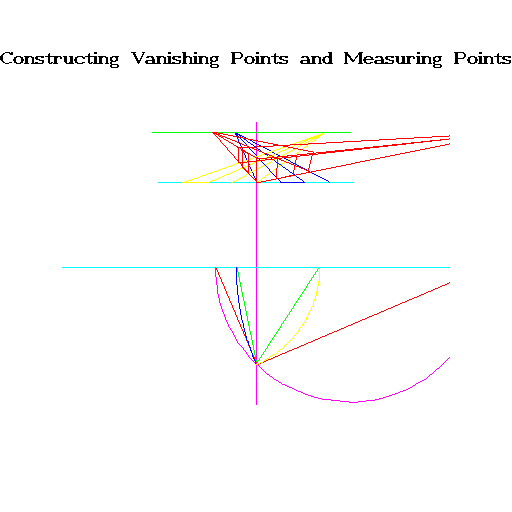MAPLE generated measuring lines viewed in perspective and their construction viewed from the top.

##Analytic Treatment of the Perspective View of a Circle

One is taught in drawing class, that circular objects in three-dimensional Euclidean Space are drawn in perspective as ellipses. The usual construction is to draw a square around the circle, and then project the perspective view of the square by finding its edges using the vanishing points and measuring points, the center by drawing the diagonals, and then sketching the projected circle by drawing it tangent to the projected square. A beginner will sometimes make the mistake of trying to make the tangency points the same as the endpoints of the axes of the ellipse, but they are not the same as seen in the p.  17 figure. But why is the image exactly the ellipse and not some other closed curve?

We shall answer this question by figuring out the equation of the image of the circle on the perspective drawing. We'll be using the methods of analytic geometry, where curves are represented by equations. Thus we shall describe a circle in three space by describing it as the locus of points satisfying certain equations. We then compute the corresponding perspective locus in terms of the Cartesian coordinates of the drawing plane. Finally, after some simplification, we will be able to recognize the curve as an ellipse.

The conic sections in the plane are given as the locus, that is the set of all points (u,v) in E2 which satisfy a quadratic equation of the form

(1.)

au2 + 2buv + cv2 + eu + fv + g = 0,

where a, b, c, d, e, f, g are constants. This can be deduced from the geometric description of the conic section as the intersection in three space of a plane with a right circular cone. All possible conic sections arise this way including degenerate ones such as lines and points and the empty set. For example if a=b=c=0 then

e u + f v + g = 0

is the equation of a line and if a=c=1, b=0, d=-2u0, e=-2v0, g=-u02-v02 then

a u2 + c v2 + e u + f v + g = (u-u0)2 + (v-v0)2 = 0

is satisfied only by one point (u,v)=(u0,v0) whereas

u2 + v2 + 1 = 0

has no real solution at all. On the other hand if the discriminant

D = a c - b2

is negative, then the conic is a hyperbola, if D=0 the conic is a parabola and if D is positive the conic is an ellipse. The easiest to see are the canonical conic curves given by the formulae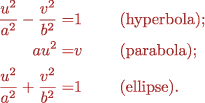Of course if a=b the ellipse is a circle.

Now let's see what a projective transformation looks like analytically. For simplicity, we assume that the set is located in front of the observer (all points of the circle satisfy y0.) Then the horizontal and vertical coordinates of the drawing plane (points which satisfy y=1) are

(2.)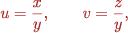where (x, y, z) runs through all points of the original set. Now suppose that we consider a circle in space with center (x0, y0, z0) and radius r and which lies on a plane not parallel to the drawing plane. By a rotation around the y-axis, we may arrange that the intersection line of the circle plane and the drawing plane is horizontal. In other words, the equation of the plane through the center of the circle sloping away from the drawing plane with slope m is given by

(3.)

z - z0 = m (y - y0).

To be able to see the circle, we require that the eyepoint (0, 0, 0) is not on the plane of the circle, which means z0 does not equal m y0 . The circle also lies on the sphere of radius r centered at (x0, y0, z0), which has the equation

(4.)

(x - x0)2 + (y - y0)2 + (z - z0)2 = r2.

The circle is the collection of points satisfying both (3.) and (4.) These are projected using (2.) to the drawing plane. By substituting (3.) into (4.),

(5.)

(x - x0)2 + (1 + m2)(y - y0)2 = r2.

We are trying to see how these equations relate u to v. Using (2.), we substitute in the equations (3.) and (5.)

v y - z0 = m (y - y0)

so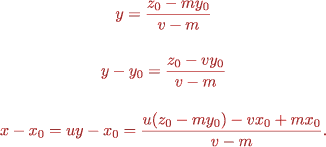Substituting into equation (5.) and multiplying by (v - m)2 yields

[u(z0 - m y0) - v x0 + m x0]2 + (1 + m2)(z0 - v y0)2 = r2(v - m)2.

Multiplying out and collecting factors of u2, uv, ... yields

(z0 - m y0)2u2 + 2 x0(z0 - m y0)u v + [x02 + (1 + m2)y02 - r2]v2 +
2 m x0(z0 - m y0)u + 2[m r2 - m x02 - (1 + m2)y0 z0]v +
[m2 x02 + (1 + m2)z02-m2r2] = 0.

Thus (u,v) satisfy a quadratic equation in the plane. The discriminant is

D= (z0 - m y0)2[x02 + (1 + m2)y02 - r2] - (z0 - m y0)2x02
= (z0 - m y0)2[(1 + m2)y02 - r2].

Since the eyepoint is not on the plane of the circle z0 - m y0 > 0. Since the circle is in front of the y=0 plane, the point (x0, 0, z0 + m y0) which is both in the y=0 plane and on the circle plane is can't be on the circle, in fact it is farther from the center than any point of the circle, hence

(1 + m2)y02 - r2 0.

Thus D > 0 and the locus is an ellipse.

Perspective view of the circle

Here is a diagram from Alberti's treatise. The square that surrounds the circle projects to a trapezoid. The circle itself projects to an ellipse which is tangent to all four sides of the trapezoid. Observe that the left and right endpoints of the axes of the ellipse where the ellipse is widest occur below the tangency points. But be careful when drawing the ellipse which is not centered on the eyepoint to centerpoint line!

##What is Projective Geometry?

The original impetus to projective geometry came from perspective drawing. Alberti's textbook Della Pittura (1435) formulated new questions that tempted mathematicians to study new questions beyond those addressed by the Greeks. If two artists make perspective drawings of the same object, their drawings will not be the same, for example because different parts of the object will be closer to each of the the two artists. But what properties of the drawings remain the same? (Diagram of Alberti's question.)

The perspective projection, which takes points X of the object which are in three space and plots them as points P on the drawing plane. Let us write this

P=f(X).

It has the property that points are mapped to points and lines to lines. However, parallel lines in three space which are not parallel to the drawing plane must be drawn to converge at their vanishing points. Thus the correspondence between lines and points in three space and lines and points on the drawing isn't perfect. Thus if L1 and L2 are parallel lines in three space then f(L1) and f(L1) are lines which intersect at V their vanishing point. However L1 and L2 don't intersect at any point. In the diagram, lines AB, CD and E'V' are parallel. Their projections A'B', C'D' intersect at a point V' which is called the vanishing point since it has no corresponding point in three space.

The solution was proposed by Girard Desargues (1591-1661) a self educated man who worked as an architect after leaving the army. His opus with the ponderous name, Broullion project d`une atteinte aux événemens des renconteres du cône avec un plan, (1693) which describes projective methods in geometry went unnoticed. Jean-Victor Poncelet (1788-1867), an engineer in Napoleon's army reworked the theory in Traité des proprietiés projectives des Figures(1822) while a prisoner of war in Russia in 1813 [KF]. This elevated Desargues work in projective geometry to one of the success stories of synthetic geometry, whose merits versus analytic geometry were being debated at the time. We sketch two theorems from projective geometry. For a more rigorous treatment, the reader should consult any of a number of texts, such as O'Hara & Ward [OW] or Wylie [WC].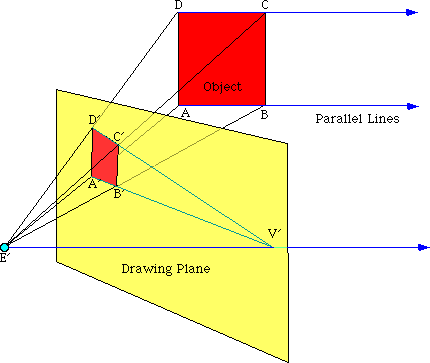To complete the correspondence, Desargues introduced ideal points, called points at infinity one for each set of parallel lines. The points at infinity don't contradict any axioms. They function as a convenience since now every pair of lines intersects at one point, the case of parallel lines does not have to be treated as an exceptional case. The following is now called Desargues' Theorem of Homologous Triangles.

Theorem. Suppose there is a point O and triangles ABC and A'B'C' in the plane or three space. If they are projectively related from the point O, that is, the triples {O, A, A'}, {O, B, B'} and {O, C, C'} are all collinear. Then the points of intersections of the corresponding sides AB and A'B', AC and A'C' and BC and B'C' (or their prolongations) are collinear. Conversely, if the three pairs of corresponding sides meet in three points which lie on one straight line, then the lines joining corresponding vertices meet at one point (are projectively related.)

The proof is easier for the case that the triangles are not coplanar. See Dörrie [HD] or Meserve [MB] for proofs.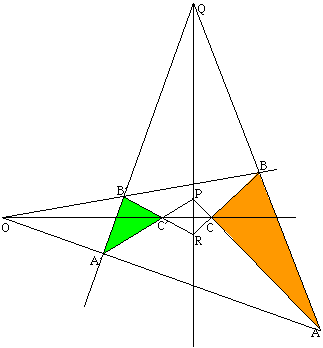Diagram of Desargues' Theorem of Homologous Triangles.

To see how we may use projective geometry directly to argue that the perspective image of a circle is an ellipse, we use a theorem due to Blaise Pascal (1623-1662). Pascal, who was urged to investigate the relationship between projectivities and conics by Desargues, published his Essai sur les Coniques when he was sixteen. Although he didn't prove the converse part, the theorem is known as Pascal's Hexagon Theorem.

Theorem. Let a hexagon be inscribed in a (nonsingular point-) conic. Then the three points of intersection of pairs of opposite sides are collinear. Conversely, if the opposite sides of a hexagon, (of which no three vertices lie on a straight line) intersect on a straight line, the six vertices lie on a non-singular point-conic.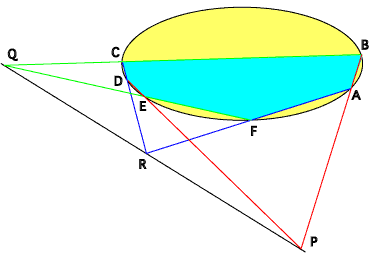Diagram for Pascal's Hexagon Theorem.

Pascal's Theorem may be used to deduce that the perspective image of a circle is an ellipse. Thus if c is the circle and f(c) is its image in a perspective drawing relative to the eyepoint O, then we have to show that if any six points A, B, C, D, E, F are chosen on f(c) so that no three of them lie on a straight line then the pairs of opposite sides intersect in collinear points. Then by the converse of Pascal's Theorem, the six points lie on a nonsingular point-conic. But since five points determine a conic, the sixth point which may be any general point of f(c) must be on the same on the conic. It follows that no matter which six points are chosen, they lie on the same conic, thus f(C) is (part of) a single point-conic. One argues that f(c) is bounded and nondegenerate thus can only be the ellipse. But the six points are in perspective correspondence to points A', B', C', D', E', F' on c which is a circle, hence a point-conic. Therefore, by Pascal's Theorem, the pairs of opposite sides (A'B' and E'D'), (B'C' and F'E'), and (C'D' and A'F') intersect at points P', Q', R' respectively, which are collinear in the plane of c. Their perspective images P, Q, R in the plane of F(c) must also be collinear since the perspective image of a line not containing O is a line. Moreover the planes OA'B', OE'D' contain the edges AB, ED, resp., since they are perspective to each other, and thus the planes intersect along the line OP'P. In other words, the point P is the intersection of the edges AB and ED. Similarly Q is the intersection of the edges BC and FE and R is the intersection of the edges CD and BF. Thus P, Q, R are collinear and we are done.

An analytic version is in the previous section Analytic Treatment of the Perspective View of a Circle.

##Computer Graphics.

Without going very deep into computer science complications, we explain something about the mathematics behind computer drawing. Computer science issues are treated, e.g. in [PP]. One of the ways that a computer renders three-dimensional object is to build up the image from little constituent pieces. The object is regarded as a collection of polygons. The visual position of each little piece is computed and the polygons are drawn one polygon at a time. The computer screen is given a Cartesian (horizontal and vertical axis) coordinate system and the polygon is drawn specifying the position of each P=(u,v) of its vertices. For example, as the three triangles red (0,0),(4,-1),(1,.5), green (4,2),(1,-.5),(4,1) and blue(4,-2),(3,1.5),(3,-1.5) are drawn, each one covers the previous ones.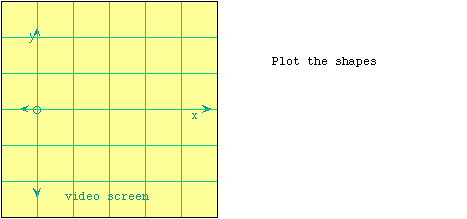If for example, we wish to draw the front elevation of the an object in space consisting of the three triangles [(0,1,0),(4,0,-1),(1,1,.5)], [(4,1,2),(1,0,-.5),(4,1,1)] and [(4,1,-2),(3,0,1.5),(3,1,-1.5)] viewed toward the +y-axis, we have to draw the triangles as before, because the projection is given by

F(x, y,z )=(x, z).

This would result in an incorrect picture because one of tips of each triangle is closer to the viewer than some one of the other triangles. e.g., the base of the first triangle (0,1,0),(1,1,.5) at y=1 is farther from the viewer than the remaining vertex (4,0,-1) at y=0. Another source of error would be if polygons in the object actually intersected. To correctly render the front elevation, the triangles have to be subdivided further into parts and the parts in front have to be drawn on top of parts in back.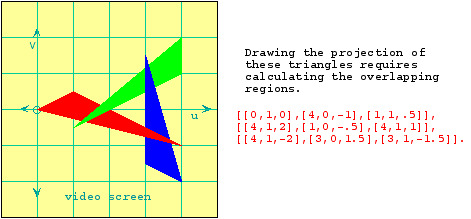The most fundamental way to depict depth in a picture is overlapping closer objects over farther ones. In general it is quite involved to decide if some part of the object can be seen or not. The simple way to deal with this is to draw all polygons of the object back to front. Some of the polygons which are in back of the object eventually get completely covered up. This is called the painter's algorithm. The way it works in our MAPLE program, first we compute the distances of each point to the eye. Then a typical distance is given for each polygon, which in our case is the distance to the nearest point. Then the polygons are sorted according to their typical distances, and are rendered back to front. Our program does not try to account for complicated overlaps or intersections so will sometimes render objects incorrectly. To illustrate the painter's algorithm, suppose we render a cube. The faces are drawn back to front, depending on the distance of each side to the viewer. In the example, the sky being farthest is drawn first, followed by the earth, the back face, the base, the sides, the top and finally the front, eventually covering up all but two sides.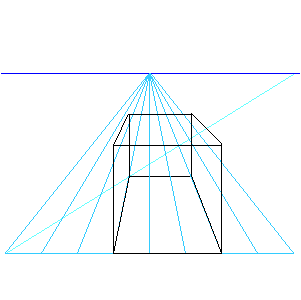Another way of rendering a three-dimensional object is called ray tracing. In ray tracing, the computer follows light rays back from the eye to a point on the object from where it figures out how intense the light is and what its color is by following back the rays which illuminate that point. This can continue for several stages. At each stage the computation accounts for surface properties like shine and color and body properties such as refractive index and transmittivity. An example of ray tracing is a rendering of the same house made by the program POV-RayTM (Persistence of VisionTM Ray-Tracer Version 3.1.) We have specified that the body be made of gray glass and be positioned on a chessboard.To get a sense of what is state of the art in ray-tracing, visit Steven Parker's website Interactive Ray Tracing -- MPEG demo at the Scientific Computing and Imaging Institute http://www.sci.utah.edu/index.html in the Graphics and Visualization group http://www.cs.utah.edu/research/areas/graphics/ in the School of Computing at the University of Utah.

##Problems.

1. Design an object to test MAPLE's 3d capability. Be sure that your object doesn't have any symmetries, so that you can tell front from back, left handed from right handed. Explore the projection for differing values of projection. (We had projection=0.7. in the runs.)
2. A right circular cone C whose center is the origin and whose axis is the z-axis satisfies the equation

F(x,y,z) = x2 + y2 - c2 z2 = 0

where c0 is constant. Suppose T:E3 --> E3 is a rigid motion, and T-1 is the inverse motion. Show that the equation of the general right circular cone T(C) is

F(T-1(X))=0.

where X = (x, y, z). Using this fact, show that the points of the intersection of the cone T(C) with the plane z = 0 also satisfy equation (1.)

3. Our rigid motions were constructed by composing rotations around the vertical axis and horizontal axes. The resulting motion maps the vertical axis to a vertical line. More generally, a rotation may occur around any axis. Find an expression for the rotation of an angle µ around an arbitrary unit vector (u1, u2, u3).
4. A projection whose rays are perpendicular to the image plane is called an orthogonal projection. It has the property that a sphere projects to the round circle. The general projection does not have this property. Suppose a test cube with side length a is projected by orthogonal projection. Consider the images of three sides incident to a corner of the cube and denote their lengths a1, a2 and a3 and let µ1, µ2 and µ3 be the angles as shown on the figure. Show that [BP p. 35]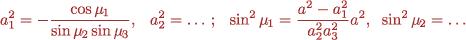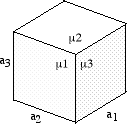5. This problem requires a little calculus. Show that if (x(t), y(t), z(t)) = (a t + x0, b t + y0, c t + z0) are points on a line that recede from the drawing plane (b 0) as t goes to infinity then the perspective transformation Fpersp.(x(t), y(t), z(t)) converges to a point depending on the direction of the line (a, b, c) and not on which line (not on (x0,y0,z0).) The limit point is the vanishing point for all parallel lines going this direction and it corresponds to the intersection of the line (a t, b t, c t) through the eyepoint and the drawing plane.
6. For one point perspective, explain why the measuring points are 45° as in the "perspective view of the circle" figure.
7. If a perspective drawing is made of a circle on the floor, which is not centered on the eyepoint-centerpoint line, which direction will it tip? Can you find a graphic construction for the major and minor axes? [Answer [EM], p. 93]

##References & Links.

BP
W. Boehm & H. Prautsch, Geometric Concepts for Geometric Design, A. K. Peters Ltd., Wellesley, 1994.
BC
C. Boyer, A history of mathematics, Princeton University Press, Princeton, 1985.
CA
A. Cole, Perspective: A visual guide to the theory and techniques from the Renaissance to Pop Art, Dorling Kindersley, Inc., New York, 1992.
DJ
J. Dauber, Mc Murray University, The art of Renaissance science. http://www.mcm.edu/academic/galileo/ars/arshtml/arch1.html
DH
H. Dörrie, Triumph der Mathematik: Hundert berühmte Probleme aus zwei Jahrtausenden mathematischer Kultur, Physica-Verlag, Würzburg, 1958; Transl. of 5th ed. 100 great problems of elementary mathematics, Dover Publications Inc., New York, 1965.
EB
B. Edwards, Drawing on the right side of the brain, 1989, Jeremy P. Tarcher, Inc., Los Angeles.
EM
M. Emrick, Computer drawing, Indianapolis Museum of Art. http://www.ima-art.org/education/schoolprograms/mathart/intro.html
EM
H. Etter & M. Malmstrom, Perspective for painters, Watson Guptill Publications, New York, 1990.
FP
I. D. Faux & M. J. Pratt, Computational geometry for design and manufacture, Ellis Horwood Ltd., Chichester, 1979, Mathematics and its applications.
GA
A. Gray, Modern differential geometry of curves and surfaces, CRC Press, Boca Raton, 1993.
IW
W. Ivins, Jr., Art & geometry: a study of space intuitions, Harvard University Press, 1946; Repub. Dover, New York, 1964.
GG
G. Gruner, Concepts in physics, notions in art, UCLA. http://www.physics.ucla.edu/class/85HC_Gruner/
KF
F. Klein, Elementarmathematik vom hoheren Standpunkte Bd. 2: Geometrie, Macmillan, New York, 1940; English transl of 3rd ed., Geometry: elementary mathematics from an advanced standpoint, Charles A. Noble, New York 1939; Repub. Dover, New York, 1948.
KM1
M. Kline, Mathematics in western culture, Oxford University Press Inc., 1953, New York.
KM2
M. Kline, Mathematical thought from ancient to modern times, Oxford University Press Inc, 1972, 232, New York.
KJ
J. Krikke, A Chinese perspective for cyberspace?, International Institute for Asian Studies Newsletter, 9, Summer 1996, http://iias.leidenuniv.nl/iiasn/iiasn9/eastasia/krikke.html
MS
S. Machlis, Drawing III, University of Idaho. http://www.art.uidaho.edu/drawing/111/lectures/lecture04.html
MB
B. Meserve, Fundamental concepts of geometry, Addison-Wesley, Reading, 1959; Repub. Dover, Mineola, 1983.
OJ
J. Oprea, Differential geometry and its applications, Prentice-Hall Inc., Upper Saddle River, 1997.
OW
C. W. O'Hara & D. R. Ward, An introduction to projective geometry, Oxford University Press, London, 1946.
PR
Robert Palais, Review of fundamental trigonometry formulas and the geometry of complex numbers and the dot product for calculus and multi-variable calculus, http://www.math.utah.edu/~cherk/ccli/bob/Rotation/Rotation.html
PP
M. Penna & R. Patterson, Projective geometry and its applications to computer graphics, Prentice Hall, Englewood cliffs, 1986.
PD
D. Pedoe, Geometry and the visual arts, St. Martin's Press Inc., NY 1976; Repub. Dover, New York, 1983.
RV
V. Rovenski, Geometry of curves and surfaces with MAPLE, 2000, Birkhäser, Boston.
SR
SC
C. Séquin, Art, math & Computers--new ways of creating pleasing shapes, Educator's TECH Exchange, Jan. 1996., http://http.cs.berkeley.edu/~sequin/edtech/edtech.html.
R. Smith, An introduction to perspective, Dorling Kindersley Ltd., -1st American ed. (The DK Art School), London, 1995.
TA
A. Treibergs, The geometry of perspective drawing: lecture notes for the talk presented to the University of Utah High School program, June 26, 2001.
WC
C. R. Wylie, Jr., Introduction to projective geometry, McGraw-Hill Inc., New York, 1970.
XX